Warm-up: Network analysis with Python | Marco Grassia

# Warm-up: Network analysis with Python

### Abstract

Python is the most widely used programming language in data analysis. In this warmup, we will introduce the most popular and powerful libraries for Network Science (i.e., graph-tool, NetworkX), for data analysis and visualization (Matplotlib, NumPy, SciPy, Pandas, Seaborn), and for Deep Learning on graphs (PyTorch Geometric).

Date
26 Jun 2022 17:30 — 18:30
Location
Catania

# Python

## What is python

Python is an interpreted, object-oriented, high-level programming language with dynamic semantics. […]

It is suitable for rapid development and for use as a “glue language” to connect various components (e.g., written in different languages).

Python is one of the most used programming languages

Its popularity can be rooted to its characteristics

• Python is fast to learn, very versatile and flexible
• It is very high-level, and complex operations can be performed with few lines of code

And to its large user-base:

• Vast assortment of libraries
• Solutions to your problems may be already available (e.g., on StackOverflow)

### Some real-world applications

• Data science (loading, processing and plotting of data)
• Scientific and Numeric computing
• Modeling and Simulation
• Web development
• Artificial Intelligence and Machine Learning
• Image and Text processing
• Scripting

## How to get python?

Using the default environment that comes with your OS is not a great idea:

• Usually older python versions are shipped
• You cannot upgrade the python version or the libraries freely
• Some functions of your OS may depend on it

You can either:

2. Use a distribution like Anaconda

### Anaconda

Anaconda is a python distribution that packs the most used libraries for data analysis, processing and visualization

Anaconda installations are managed through the conda package manager

Anaconda “distribution” is free and open sourceAnaconda distribution

# Python virtual environments

A virtual environment is a Python environment such that the Python interpreter, libraries and scripts installed into it are isolated from those installed in other virtual environments

Environments are used to freeze specific interpreter and libraries versions for your projects

If you start a new project and need newer libraries, just create a new environment

You won’t have to worry about breaking the other projects

#### Environment creation

conda create –name <ENV_NAME> [<PACKAGES_LIST>] [–channel ]

You can also specify additional channels to search for packages (in order)

Example:

conda create –name gt python=3.9 graph-tool pytorch torchvision torchaudio cudatoolkit=11.3 pyg seaborn numpy scipy matplotlib jupyter -c pyg -c pytorch -c nvidia -c anaconda -c conda-forge

#### Switch environment

conda activate <ENV_NAME>

Example

conda activate gt

Full documentation

## Data analysis and visualization libraries

• NumPy
• SciPy
• Matplotlib
• Seaborn
• Pandas

These libraries are general, and can be used also in Network Analysis

## Network analysis and visualization libraries

• graph-tool
• PyTorch Geometric (PyG)

## NumPy

NumPy is the fundamental package for scientific computing in Python.

NumPy offers new data structures:

• Multidimensional array objects (the ndarray)
• Various derived objects (like masked arrays)

And also a vast assortment of functions:

• Mathematical functions (arithmetic, trigonometric, hyperbolic, rounding, …)
• Sorting, searching, and counting
• Operations on Sets
• Input and output
• Fast Fourier Transform
• Complex numbers handing
• (pseudo) random number generation

NumPy is fast:

• Its core is written in C/C++
• Arrays are stored in contiguous memory locations

It also offers tools for integrating C/C++ code

Many libraries are built on top of NumPy’s arrays and functions.

#### The ndarray

Mono-dimensionalMulti-dimensional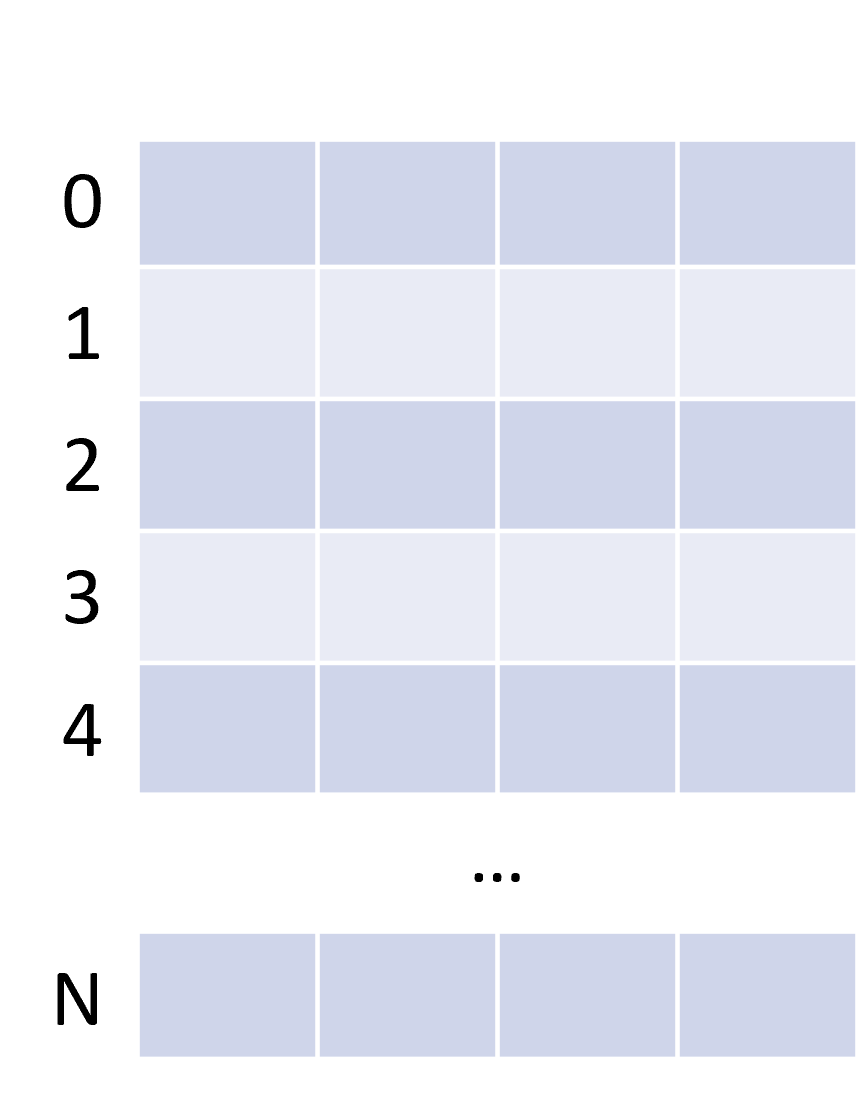NumPy documentation

 1  import numpy as np 
 1  np.random.rand(3) 
array([0.23949294, 0.49364534, 0.10055484])

 1  np.random.rand(1, 3) 
array([[0.45292492, 0.32975629, 0.53797728]])

 1  np.random.randint(10, size=(2, 2, 2)) 
array([[[9, 1],
[9, 9]],

[[5, 7],
[3, 3]]])


## SciPy

SciPy is a collection of mathematical algorithms and convenience functions built on the NumPy library

SciPy is written in C and Fortran, and provides:

• Algorithms for optimization, integration, interpolation, eigenvalue problems, algebraic equations, differential equations, statistics, etc.
• Specialized data structures, such as sparse matrices and k-dimensional trees
• Tools for the interactive Python sessions

SciPy’s main subpackages include:

• Data clustering algorithms

• Physical and mathematical constants

• Fast Fourier Transform routines

• Integration and ordinary differential equation solvers

• Linear algebra

• N-dimensional image processing

• Optimization and root-finding routines

• Signal processing

• Sparse matrices and associated routines

• Spatial data structures and algorithms

• Statistical distributions and functions

 1  import scipy as sp 

SciPy documentation

### Sparse matrices

There are many sparse matrices implementations, each optimized for different operations.

For instance:

• Coordinate (COO)
• Linked List Matrix (LIL)
• Compressed Sparse Row (CSR)
• Compressed Sparse Column (CSC)

Check this nice tutorial for more! Sparse matrices tutorial

## Pandas

pandas allows easy data organization, filtering, analysis and plotting

pandas provides data structures for “relational” or “labeled” data, for instance:

• Tabular data with heterogeneously-typed columns, as in an Excel spreadsheet
• Ordered and unordered time series data
• Arbitrary matrix data (even heterogeneous typed) with row and column labels

The two primary data structures provided are the:

• Series (1-dimensional)
• DataFrame (2-dimensional)
SeriesDataFrame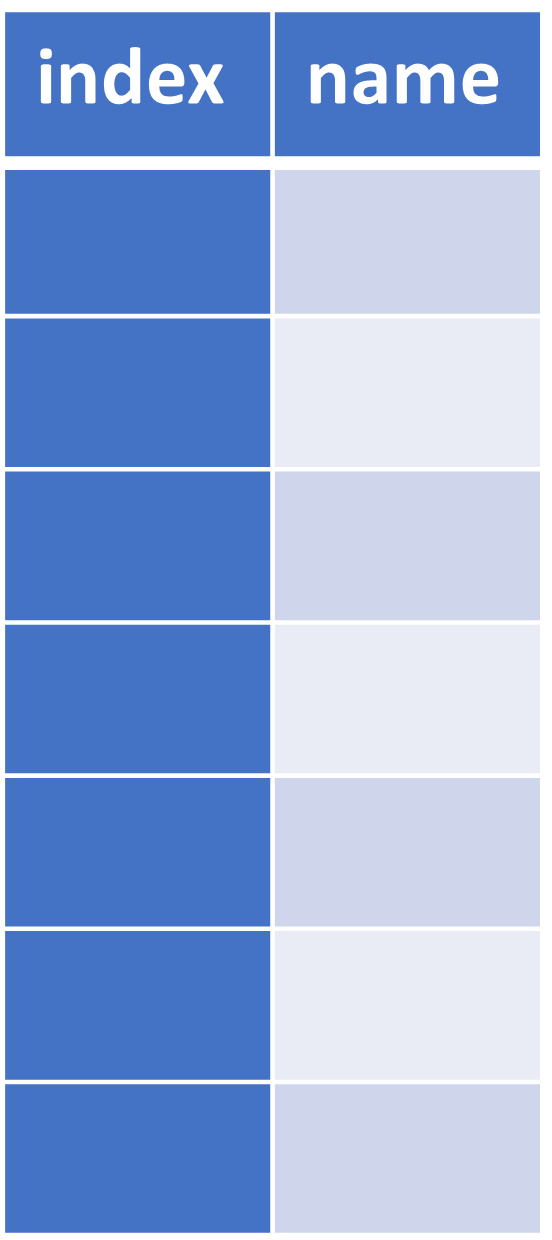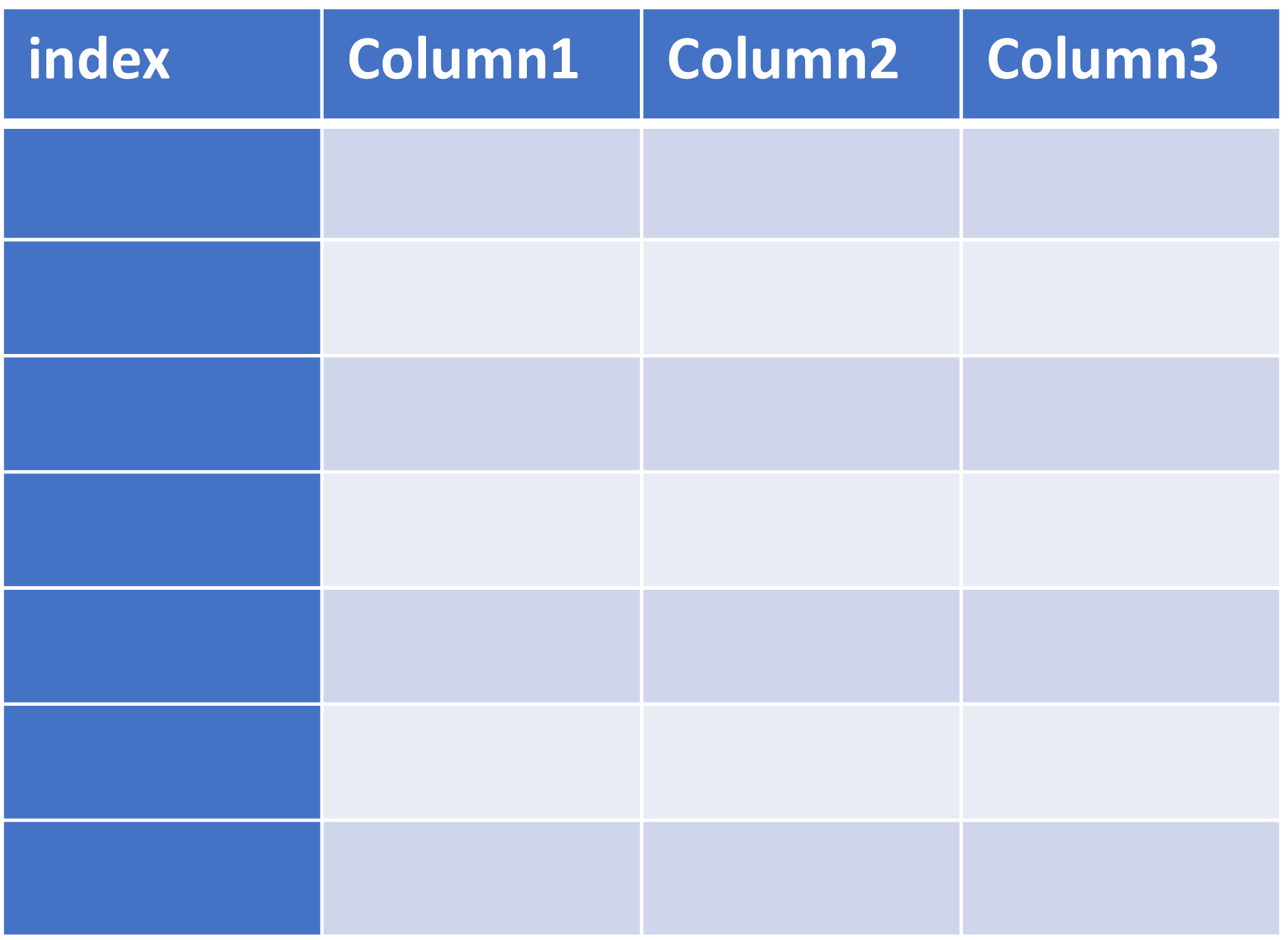These structures heavily rely on NumPy and its arrays

pandas integrates well with other libraries built on top of NumPy

#### Supported file formas

pandas can recover data from/store data to SQL databases, Excel, CSVs…1  import pandas as pd 

pandas documentation

#### DataFrame example

Penguins example dataset from the Seaborn package

 1 2  penguins = sns.load_dataset("penguins") display(penguins) 

speciesislandbill_length_mmbill_depth_mmflipper_length_mmbody_mass_gsex
........................
339GentooBiscoeNaNNaNNaNNaNNaN
340GentooBiscoe46.814.3215.04850.0Female
341GentooBiscoe50.415.7222.05750.0Male
342GentooBiscoe45.214.8212.05200.0Female
343GentooBiscoe49.916.1213.05400.0Male

344 rows × 7 columns

##### Series of the species
 1  penguins["species"] 
0      Adelie
...
339    Gentoo
340    Gentoo
341    Gentoo
342    Gentoo
343    Gentoo
Name: species, Length: 344, dtype: object

##### Unique species
 1  penguins["species"].unique() 
array(['Adelie', 'Chinstrap', 'Gentoo'], dtype=object)

##### Average bill length
 1  penguins["bill_length_mm"].mean() 
43.9219298245614

##### Standard deviation of the bill length
 1  penguins["bill_length_mm"].std() 
5.4595837139265315

##### Data filtering for male penguins
 1  penguins["sex"] == "Male" 
0       True
1      False
2      False
3      False
4      False
...
339    False
340    False
341     True
342    False
343     True
Name: sex, Length: 344, dtype: bool

 1 2 3  penguins.loc[ # .loc property penguins["sex"] == "Male" # Row filter (boolean) ] 

speciesislandbill_length_mmbill_depth_mmflipper_length_mmbody_mass_gsex
........................
333GentooBiscoe51.516.3230.05500.0Male
335GentooBiscoe55.116.0230.05850.0Male
337GentooBiscoe48.816.2222.06000.0Male
341GentooBiscoe50.415.7222.05750.0Male
343GentooBiscoe49.916.1213.05400.0Male

168 rows × 7 columns

##### Average bill length for male penguins
 1 2 3  penguins.loc[ penguins["sex"] == "Male", # Mask (row filter) "bill_length_mm", # Column filter ].mean() 
45.85476190476191

##### Average bill length and weight for female penguins
 1 2 3  penguins.loc[penguins["sex"] == "Female", # Mask (row filter) ["bill_length_mm", "body_mass_g"] # Column filter ].mean() 
bill_length_mm      42.096970
body_mass_g       3862.272727
dtype: float64


## Matplotlib

Matplotlib is a comprehensive library for creating static, animated, and interactive visualizations in Python

Matplotlib documentation

### Some plot examples

From the Matplotlib gallery

#### Line plots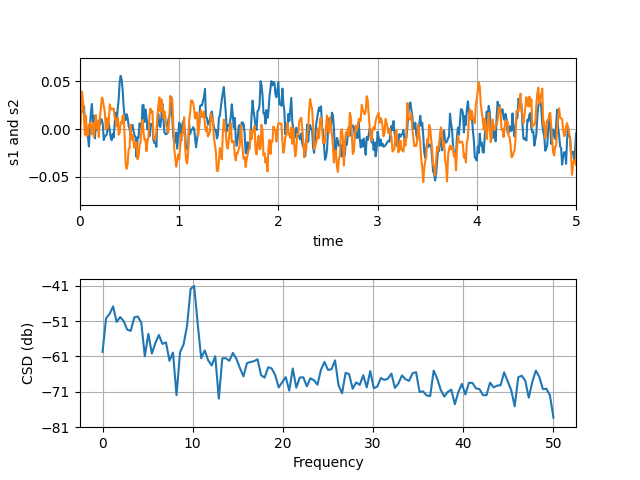Source

#### Scatterplots and histograms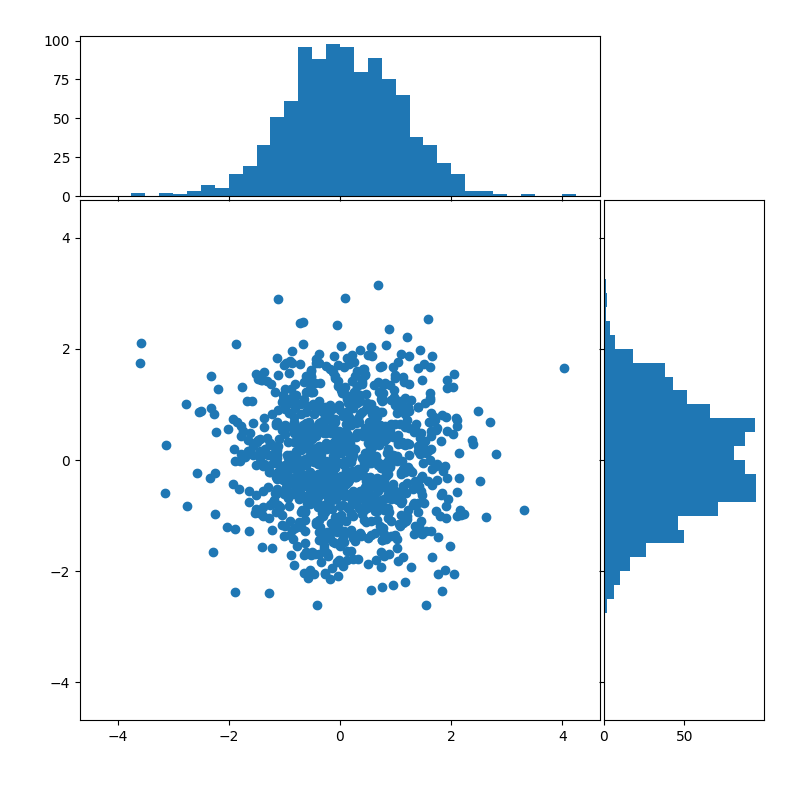Source

#### Barplots

##### Simple barplot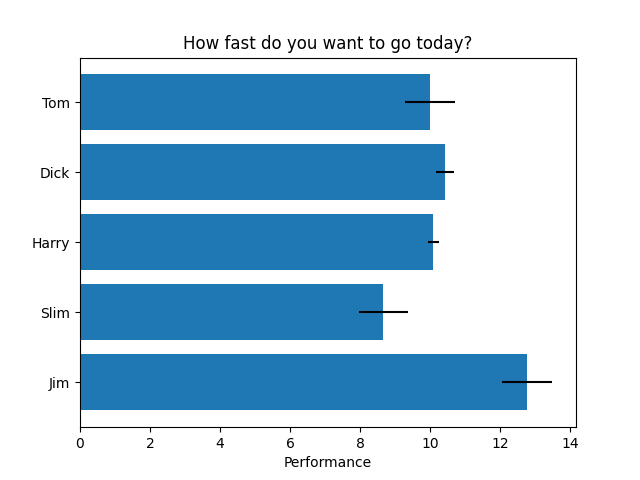Source

##### Stacked barplotSource

##### Grouped barplot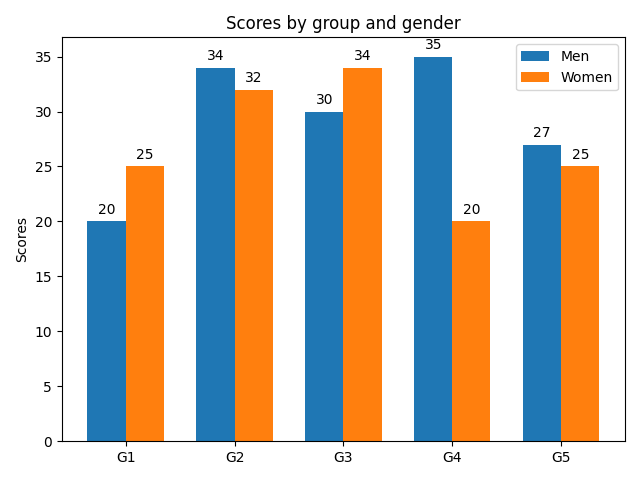Source

#### Horizontal bar chartSource

### (Nested) pie charts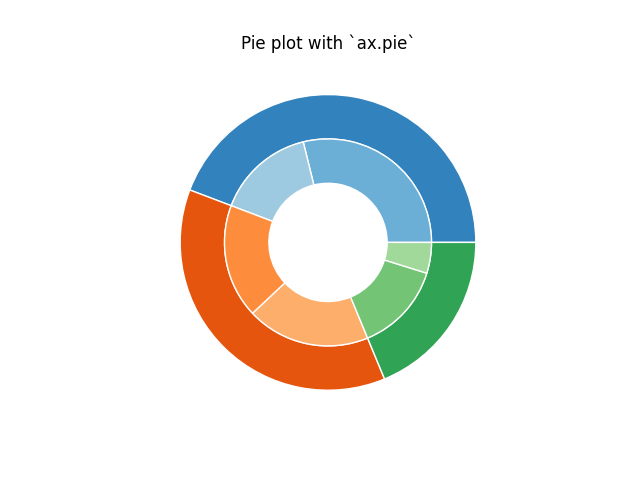Source

#### HeatmapsSource

#### Violin and box plots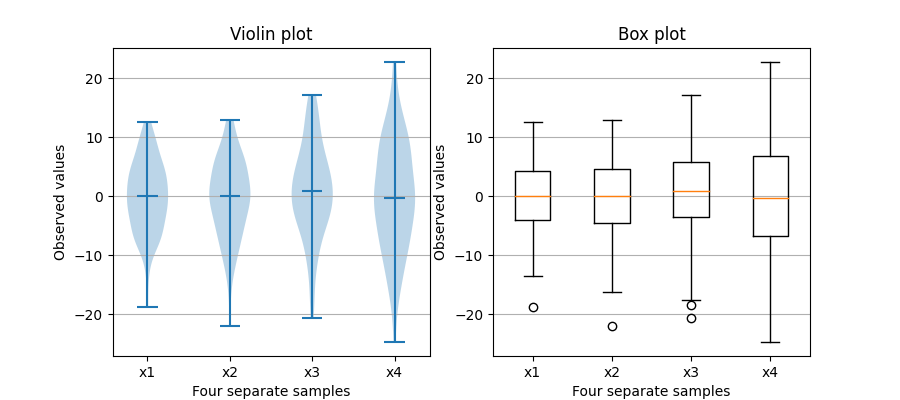Source

#### Stackplots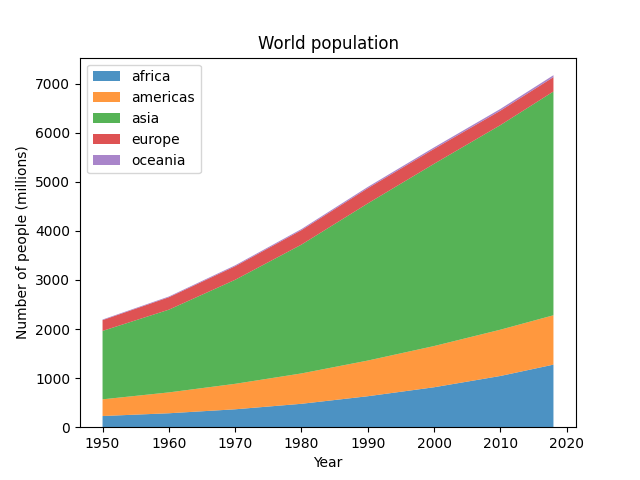Source

#### … and many more

 1  import matplotlib.pyplot as plt 

## Seaborn

Seaborn is a library for making statistical graphics in Python

Thanks to its high-level interface, it makes plotting very complex figures easy

Seaborn builds on top of matplotlib and integrates closely with pandas data structures

 1  import seaborn as sns 

It provides helpers to improve how all matplotlib plots look:

• Theme and style
• Colors (even colorblind palettes)
• Scaling, to quickly switch between presentation contexts (e.g., plot, poster and talk)
 1  sns.reset_defaults() 
 1  plt.plot(range(10), range(10)) 
[<matplotlib.lines.Line2D at 0x7f1ed75972b0>]1 2 3 4 5 6 7 8 9 10 11 12 13 14  sns.set_theme(context="talk", style="ticks", palette="deep", font="sans-serif", # font_scale=1, color_codes=True, rc={ 'figure.facecolor': 'white' # 'figure.figsize': (10, 6), # "text.usetex": True, # "font.family": "sans-serif", }, ) 
 1 2  plt.plot(range(10), range(10)) sns.despine()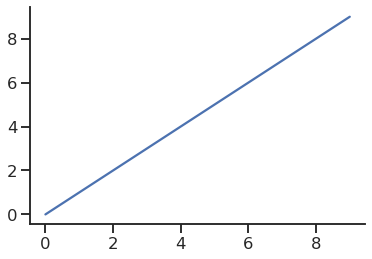Seaborn’s FacetGrid offers a convenient way to visualize multiple plots in grids

They can be drawn with up to three dimensions: rows, columns and hue

Tutorial: Building structured multi-plot grids

 1  penguins.head() 

speciesislandbill_length_mmbill_depth_mmflipper_length_mmbody_mass_gsex
 1 2 3 4 5 6 7  g = sns.relplot(data=penguins, x="flipper_length_mm", y="bill_length_mm", col="sex", hue="body_mass_g" ) g.set_axis_labels("Flipper length (mm)", "Bill length (mm)") 
<seaborn.axisgrid.FacetGrid at 0x7f1ed7597a90>1 2 3 4 5 6 7 8  g = sns.relplot(data=penguins, x="flipper_length_mm", y="bill_length_mm", row="sex", col="species", hue="body_mass_g" ) g.set_axis_labels("Flipper length (mm)", "Bill length (mm)") 
<seaborn.axisgrid.FacetGrid at 0x7f1ed73a5f10>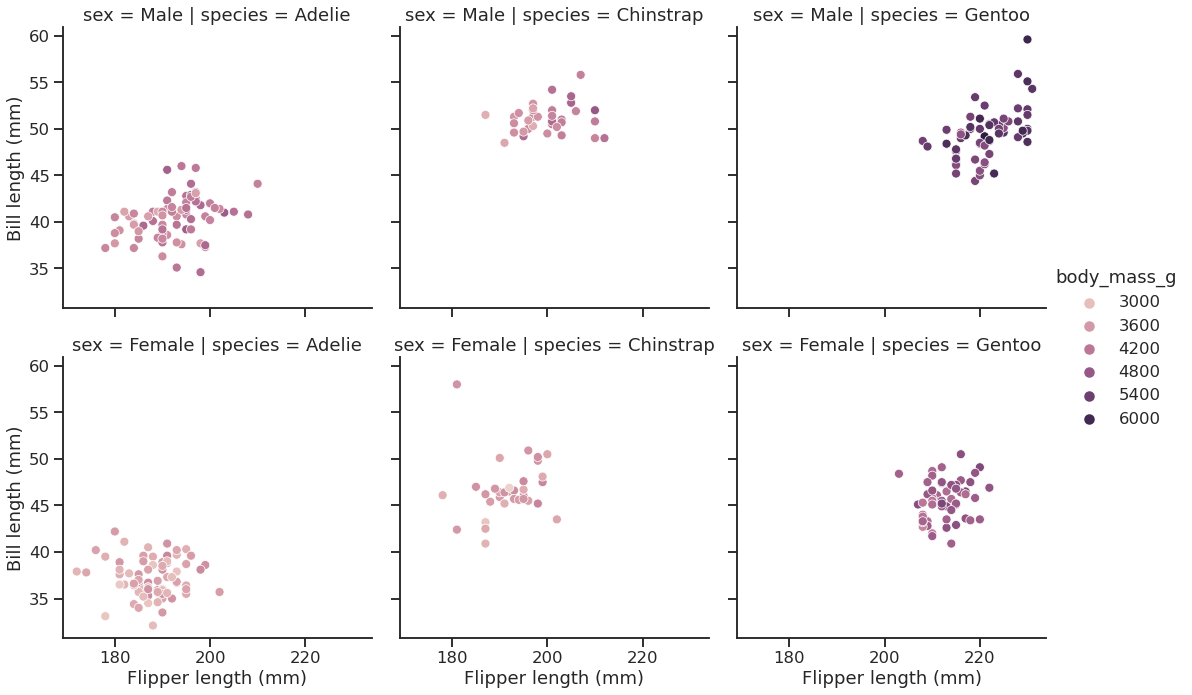Seaborn documentation

### Some more plot examples

#### Smooth kernel density with marginal histograms (source)

 1 2 3 4 5  g = sns.JointGrid(data=penguins, x="body_mass_g", y="bill_depth_mm", space=0) g.plot_joint(sns.kdeplot, fill=True, clip=((2200, 6800), (10, 25)), thresh=0, levels=100, cmap="rocket") g.plot_marginals(sns.histplot, color="#03051A", alpha=1, bins=25) 
<seaborn.axisgrid.JointGrid at 0x7f1ed5671ca0>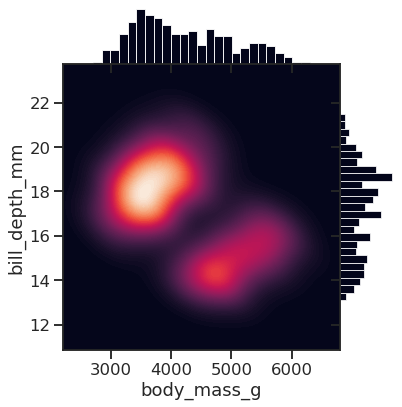#### Joint and marginal histograms

Source

  1 2 3 4 5 6 7 8 9 10 11 12 13 14  g = sns.JointGrid(data=planets, x="year", y="distance", marginal_ticks=True) # Set a log scaling on the y axis g.ax_joint.set(yscale="log") # Create an inset legend for the histogram colorbar cax = g.figure.add_axes([.15, .55, .02, .2]) # Add the joint and marginal histogram plots g.plot_joint( sns.histplot, discrete=(True, False), cmap="light:#03012d", pmax=.8, cbar=True, cbar_ax=cax ) g.plot_marginals(sns.histplot, element="step", color="#03012d") 
<seaborn.axisgrid.JointGrid at 0x7f1ed4503c70>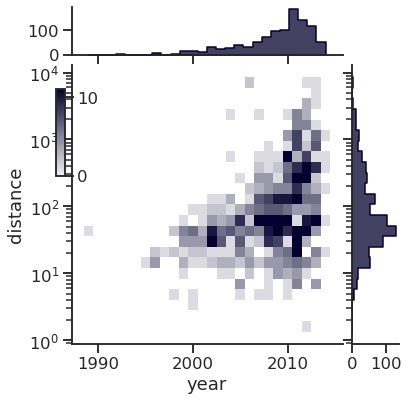#### Custom projections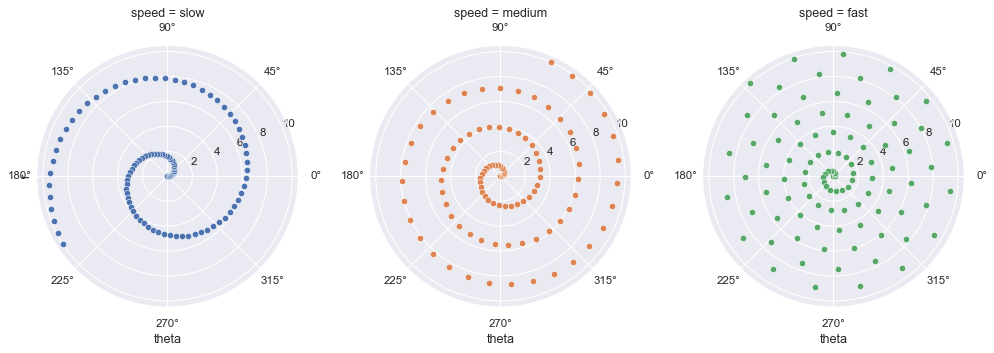Source

#### Discovering structure in heatmap dataSource

#### Bivariate plot with multiple elements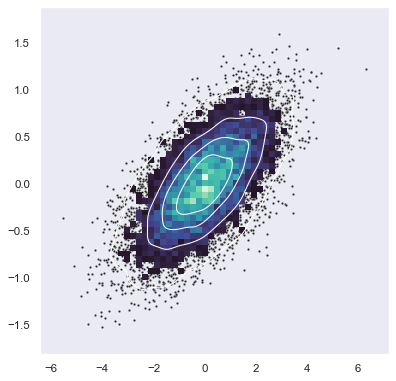Source

# Network Analysis with Python

Three main libraries:

• graph-tool
• networkx
• python-igraph

## graph-tool

graph-tool is a graph analysis library for Python

It provides the Graph data structure, and various algorithms

It is mostly written in C++, and based on the Boost Graph Library

It supports multithreading and it is fairly easy to extend

Built in algorithms:

• Topology analysis tools
• Centrality-related algorithms
• Clustering coefficient (transitivity) algorithms
• Correlation algorithms, like the assortativity
• Dynamical processes (e.g., SIR, SIS, …)
• Graph drawing tools
• Random graph generation
• Statistical inference of generative network models
• Spectral properties computation

Documentation

 1  import graph_tool.all as gt 

Performance comparison (source: graph-tool.skewed.de/)

Single-source shortest path0.0023 s0.0022 s0.0092 s0.25 s
Global clustering0.011 s0.025 s0.027 s7.94 s
PageRank0.0052 s0.022 s0.072 s1.54 s
K-core0.0033 s0.0036 s0.0098 s0.72 s
Minimum spanning tree0.0073 s0.0072 s0.026 s0.64 s
Betweenness102 s (~1.7 mins)331 s (~5.5 mins)198 s (vertex) + 439 s (edge) (~ 10.6 mins)10297 s (vertex) 13913 s (edge) (~6.7 hours)

## How to load a network

1. Choose a graph analysis library. The right one mostly depends on your needs (e.g., functions, performance, etc.)

In this warm-up, we will use graph-tool.

2. To load the network, we need to use the right loader function, which depends on the file format

### File formats

Many ways to represent and store graphs.

The most popular ones are:

• edgelist
• GraphML

For more about file types, check the NetworkX documentation

### Edgelist (.el, .edge, …)

As the name suggests, it is a list of node pairs (source, target) and edge properties (if any). Edgelists cannot store any information about the nodes, or about the graph (not even about the directedness)

Values may be separated by commas, spaces, tabs, etc. Comments may be supported by the reader function.

Example file:

# source, target, weight
0,1,1
0,2,2
0,3,2
0,4,1
0,5,1
0,6,1
1,18,1
1,3,1
1,4,2
2,0,1
2,25,1
#...


### GraphML (.graphml, .xml)

Flexible format based on XML.

It can store hierarchical graphs, information (i.e., attributes or properties) about the graph, the nodes and the edges.

Main drawback: heavy disk usage (space, and I\O time)

Example of the file:

  1 2 3 4 5 6 7 8 9 10 11 12 13 14 15 16 17 18 19 20 21 22 23 24 25 26 27 28 29 30 31 32 33 34 35 36 37 38 39 40 41 42 43 44 45 46 47 48 49 50 51   ['J. S. Coleman. "Introduction to Mathematical Sociology." London Free Press Glencoe (1964), http://www.abebooks.com/Introduction-Mathematical-Sociology-COLEMAN-James-S/189127582/bd'] A network of friendships among male students in a small high school in Illinois from 1958. An arc points from student i to student j if i named j as a friend, in either of two identical surveys (from Fall and Spring semesters). Edge weights are the number of surveys in which the friendship was named. 0.92308158331278289, 12.186082864409657 1.2629064355495019, 12.213213242633238 1.1082744694986855, 12.190211909578192 1 2 2 1 

# How to load a network

1. Choose a graph analysis library. The right one mostly depends on your needs (e.g., features, performance, etc.)

In this warm-up, we will use graph-tool.

2. To load the network, we need to use the right loader function, which depends on the file format

3. After identifying the file format and the right loader function, we load the network

 1 2 3  g = gt.load_graph("highschool.graphml") display(g) 
<Graph object, directed, with 70 vertices and 366 edges, 1 internal vertex property, 1 internal edge property, 7 internal graph properties, at 0x7f1ed41879d0>

 1  display(g.graph_properties) 
{'citation': <GraphPropertyMap object with value type 'string', for Graph 0x7f1ed41879d0, at 0x7f1ed4187220>, 'description': <GraphPropertyMap object with value type 'string', for Graph 0x7f1ed41879d0, at 0x7f1ed4187130>, 'konect_meta': <GraphPropertyMap object with value type 'string', for Graph 0x7f1ed41879d0, at 0x7f1ed4187070>, 'konect_readme': <GraphPropertyMap object with value type 'string', for Graph 0x7f1ed41879d0, at 0x7f1ed41b80a0>, 'name': <GraphPropertyMap object with value type 'string', for Graph 0x7f1ed41879d0, at 0x7f1ed4225070>, 'tags': <GraphPropertyMap object with value type 'vector<string>', for Graph 0x7f1ed41879d0, at 0x7f1ed4225040>, 'url': <GraphPropertyMap object with value type 'string', for Graph 0x7f1ed41879d0, at 0x7f1ed4256d00>}

 1  display(g.vertex_properties) 
{'_pos': <VertexPropertyMap object with value type 'vector<double>', for Graph 0x7f1ed41879d0, at 0x7f1ed4187730>}

 1  display(g.edge_properties) 
{'weight': <EdgePropertyMap object with value type 'int16_t', for Graph 0x7f1ed41879d0, at 0x7f1ed4291130>}


## Some network analysis

#### Get the number of nodes

 1 2  number_of_nodes = g.num_vertices() display(f"Number of nodes: {number_of_nodes}") 
'Number of nodes: 70'


#### Get the number of edges

 1 2  number_of_edges = g.num_edges() display(f"Number of edges: {number_of_edges}") 
'Number of edges: 366'


#### Get the in and out degrees

 1  in_degree = g.get_in_degrees(g.get_vertices(), eweight=None) 
 1 2 3  average_in_degree = np.mean(in_degree) display("Average in degree", average_in_degree) 
'Average in degree'

5.228571428571429

 1  out_degree = g.get_out_degrees(g.get_vertices(), eweight=None) 
 1 2  average_out_degree = np.mean(out_degree) display("Average out degree", average_out_degree) 
'Average out degree'

5.228571428571429


#### In-degree distribution

 1 2 3 4 5  p = plt.hist(in_degree) plt.ylabel("Count") plt.xlabel("In-degree") sns.despine()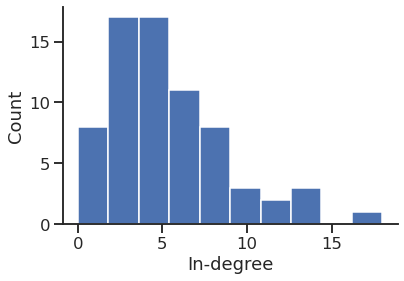1 2 3 4 5 6  p = sns.histplot(in_degree, stat="count", discrete=True, ) p.set_xlabel("In-degree") sns.despine()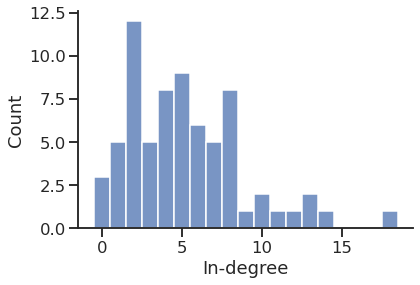1 2 3 4 5 6  p = sns.histplot(in_degree, stat="percent", discrete=True, ) p.set_xlabel("In-degree") sns.despine()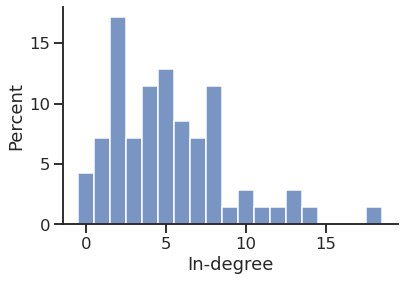1 2 3 4 5 6  sns.histplot(in_degree, stat="frequency", discrete=True, ) plt.xlabel("In-degree") sns.despine()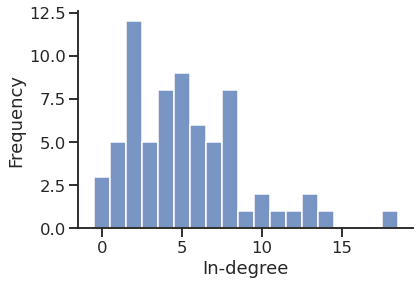1 2 3 4 5 6 7 8 9 10 11 12 13 14 15 16 17  sns.histplot(in_degree, stat="percent", discrete=True, label="In-degree", legend=True, ) sns.histplot(out_degree, stat="percent", discrete=True, label="Out-degree", legend=True, ) plt.xlabel("Degree") plt.legend() sns.despine()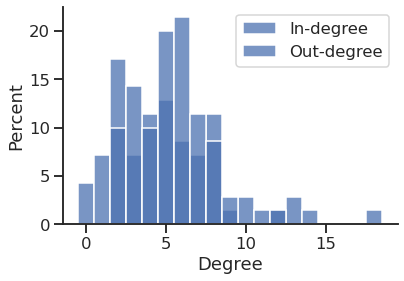1 2 3  cmap = sns.color_palette("deep", n_colors=2) cmap 

  1 2 3 4 5 6 7 8 9 10 11 12 13 14 15 16 17 18 19 20 21  sns.histplot(in_degree, stat="probability", discrete=True, label="In-degree", legend=True, color=cmap, alpha=0.6, ) sns.histplot(out_degree, stat="probability", discrete=True, label="Out-degree", legend=True, color=cmap, alpha=0.6, ) plt.title("Degree distribution") plt.xlabel("Degree") plt.legend() sns.despine()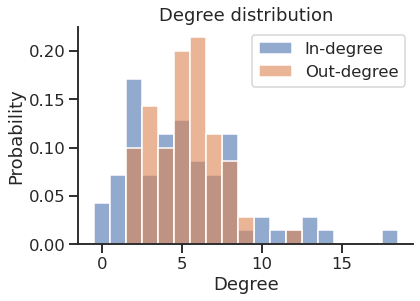#### Get the in and out strength

 1 2 3 4 5  weight = g.edge_properties["weight"] in_strength = g.get_in_degrees(g.get_vertices(), eweight=weight) out_strength = g.get_out_degrees(g.get_vertices(), eweight=weight) 
  1 2 3 4 5 6 7 8 9 10 11 12 13 14 15 16 17 18 19 20 21 22  sns.histplot(in_strength, stat="probability", discrete=False, label="In-strength", legend=True, color=cmap, alpha=0.6, ) sns.histplot(out_strength, stat="probability", discrete=False, label="Out-strength", legend=True, color=cmap, alpha=0.6, ) plt.title("Strength distribution") plt.xlabel("Strength") plt.legend() sns.despine()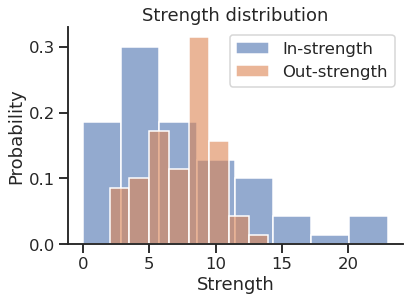### Store the values as a DataFrame

  1 2 3 4 5 6 7 8 9 10 11  df = pd.DataFrame( data={ ("Degree", "In"): in_degree, ("Degree", "Out"): out_degree, ("Strength", "In"): in_strength, ("Strength", "Out"): out_strength, }, ) df.head() 

DegreeStrength
InOutInOut
02628
12334
22435
3126199
4135219

### … and plot the DF using Seaborn

 1 2 3  melted_df = pd.melt(df, var_name=["Kind", "Direction"], value_name="Value") melted_df 

KindDirectionValue
0DegreeIn2.0
1DegreeIn2.0
2DegreeIn2.0
3DegreeIn12.0
4DegreeIn13.0
............
275StrengthOut7.0
276StrengthOut10.0
277StrengthOut10.0
278StrengthOut4.0
279StrengthOut5.0

280 rows × 3 columns

 1 2 3 4 5 6 7  facet = sns.displot(melted_df, x="Value", kind="hist", row="Kind", col="Direction", hue="Direction", )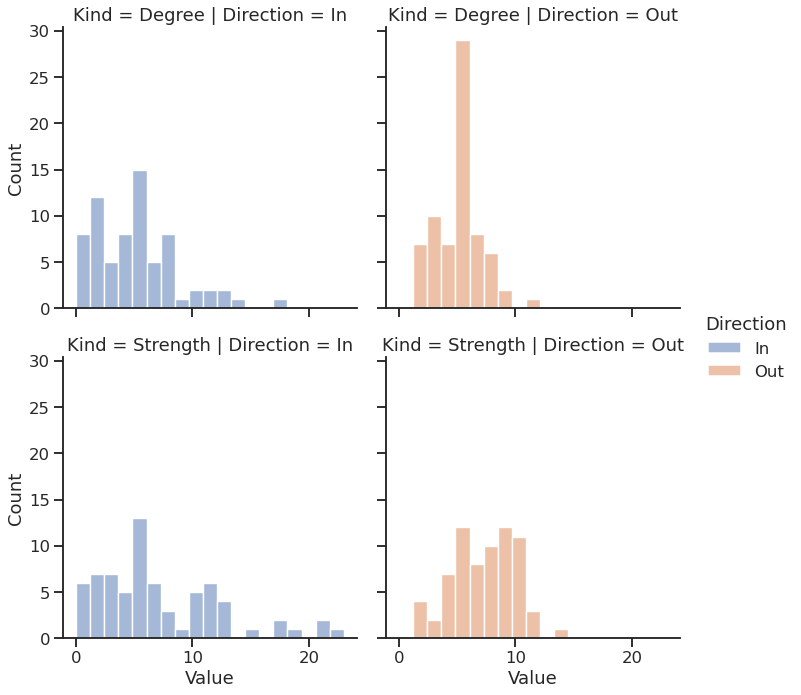1 2 3 4 5 6 7 8 9 10 11  melted_df["Kind"] = melted_df["Kind"].apply(str.lower) facet = sns.displot(melted_df, x="Value", kind="hist", row="Kind", col="Direction", hue="Direction", ) facet.set_titles(template="{col_name}-{row_name}") 
<seaborn.axisgrid.FacetGrid at 0x7f1ecf5c6610>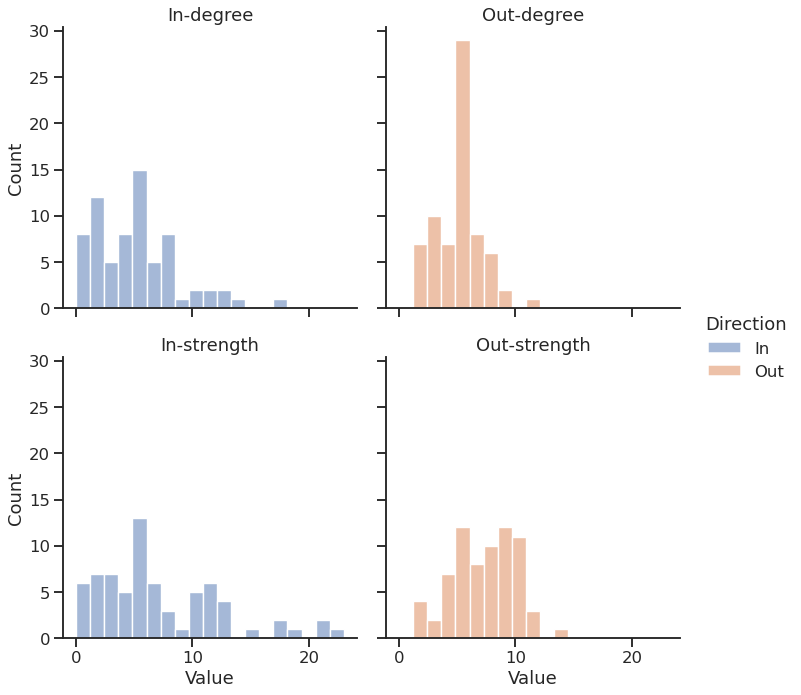## Graph visualization

#### 1. Compute the node layout

 1  pos = gt.fruchterman_reingold_layout(g, n_iter=1000) 

#### 2. Plot the network

 1 2 3  gt.graph_draw(g, pos=pos, bg_color="#111", )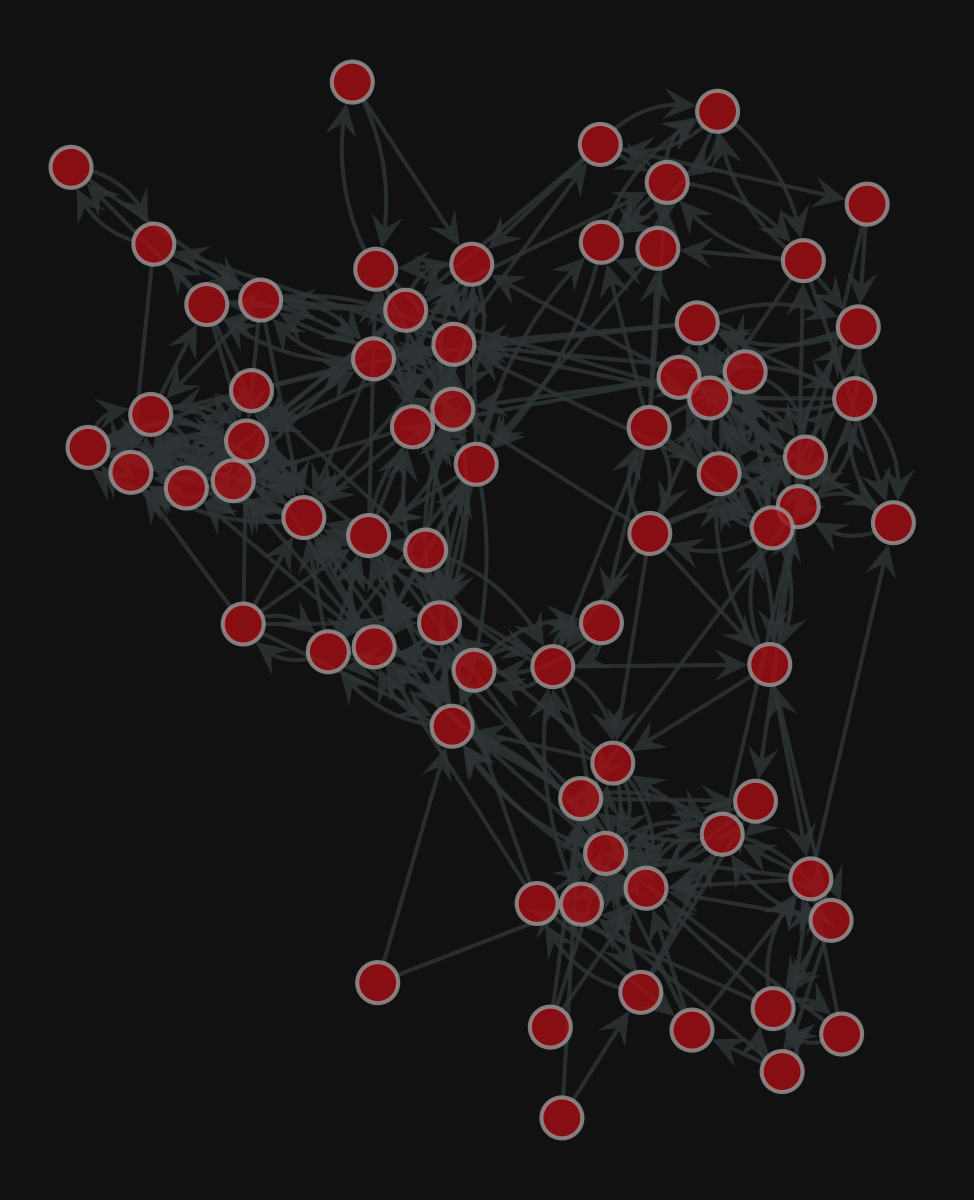<VertexPropertyMap object with value type 'vector<double>', for Graph 0x7f1ed41879d0, at 0x7f1ed41312b0>


#### Add the edge weight

 1 2 3 4 5  gt.graph_draw(g, pos=pos, edge_pen_width=g.edge_properties["weight"], bg_color="#111", )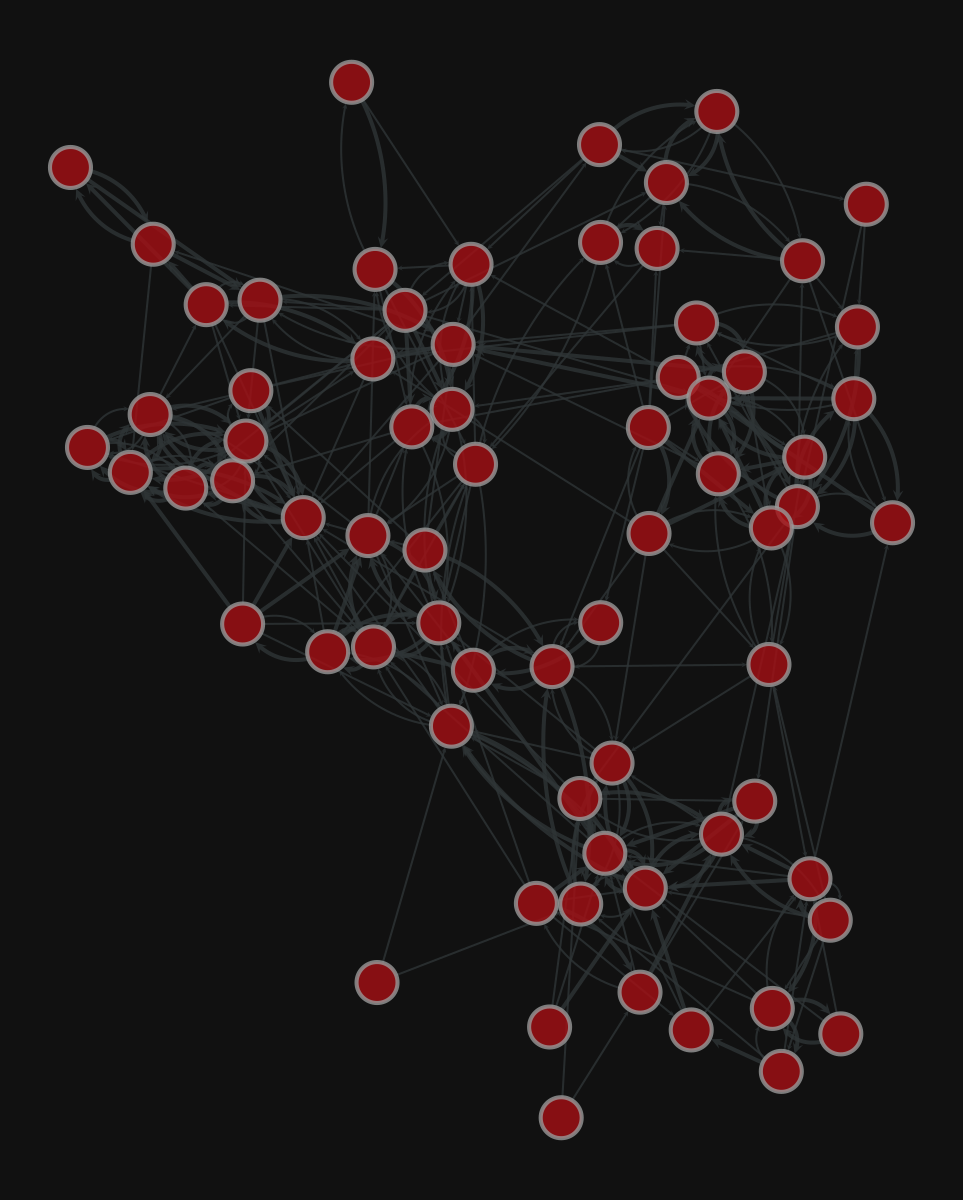<VertexPropertyMap object with value type 'vector<double>', for Graph 0x7f1ed41879d0, at 0x7f1ecf79e910>


#### More layouts

 1 2 3 4 5 6  pos = gt.sfdp_layout(g) gt.graph_draw(g, pos=pos, edge_pen_width=g.edge_properties["weight"], bg_color="#111", )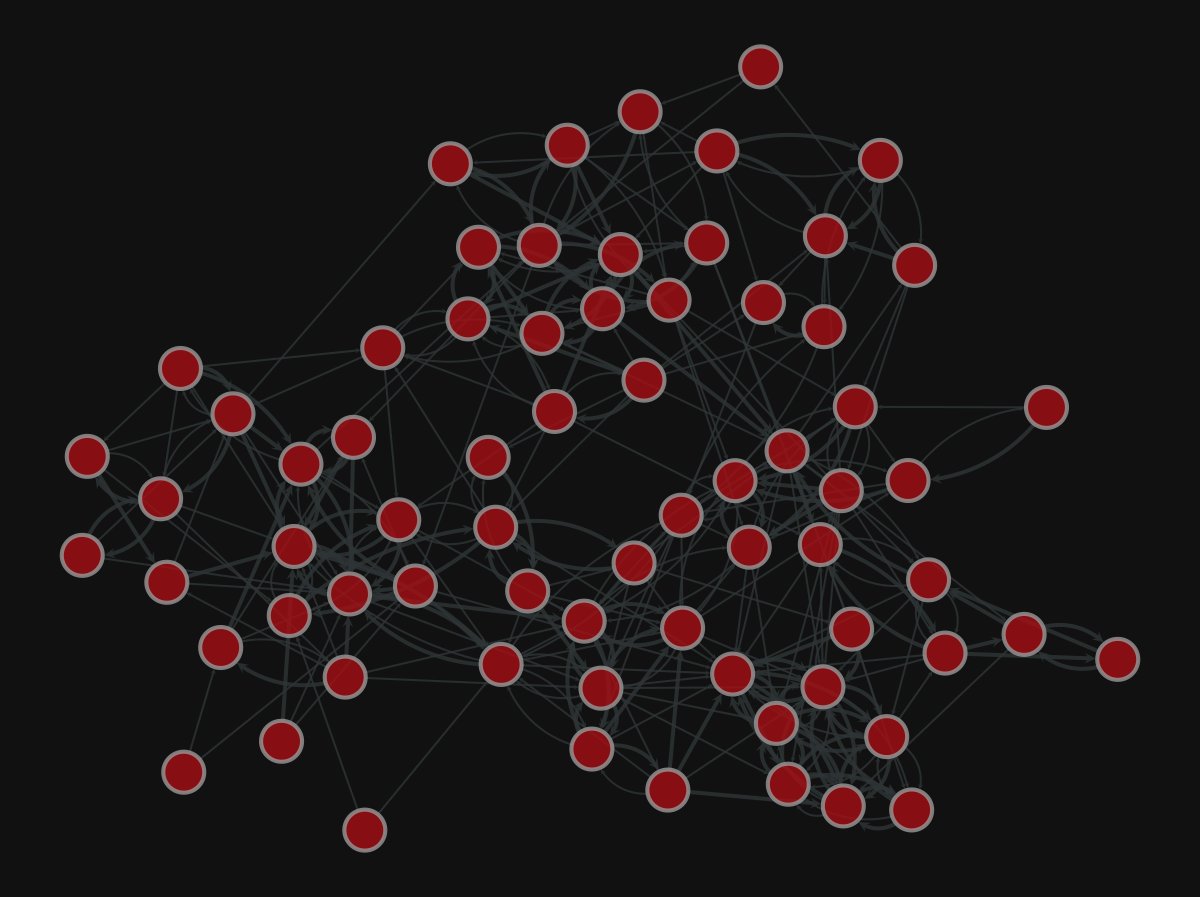<VertexPropertyMap object with value type 'vector<double>', for Graph 0x7f1ed41879d0, at 0x7f1ecf79eca0>

 1 2 3 4 5 6  pos = gt.arf_layout(g) gt.graph_draw(g, pos=pos, bg_color="#111", )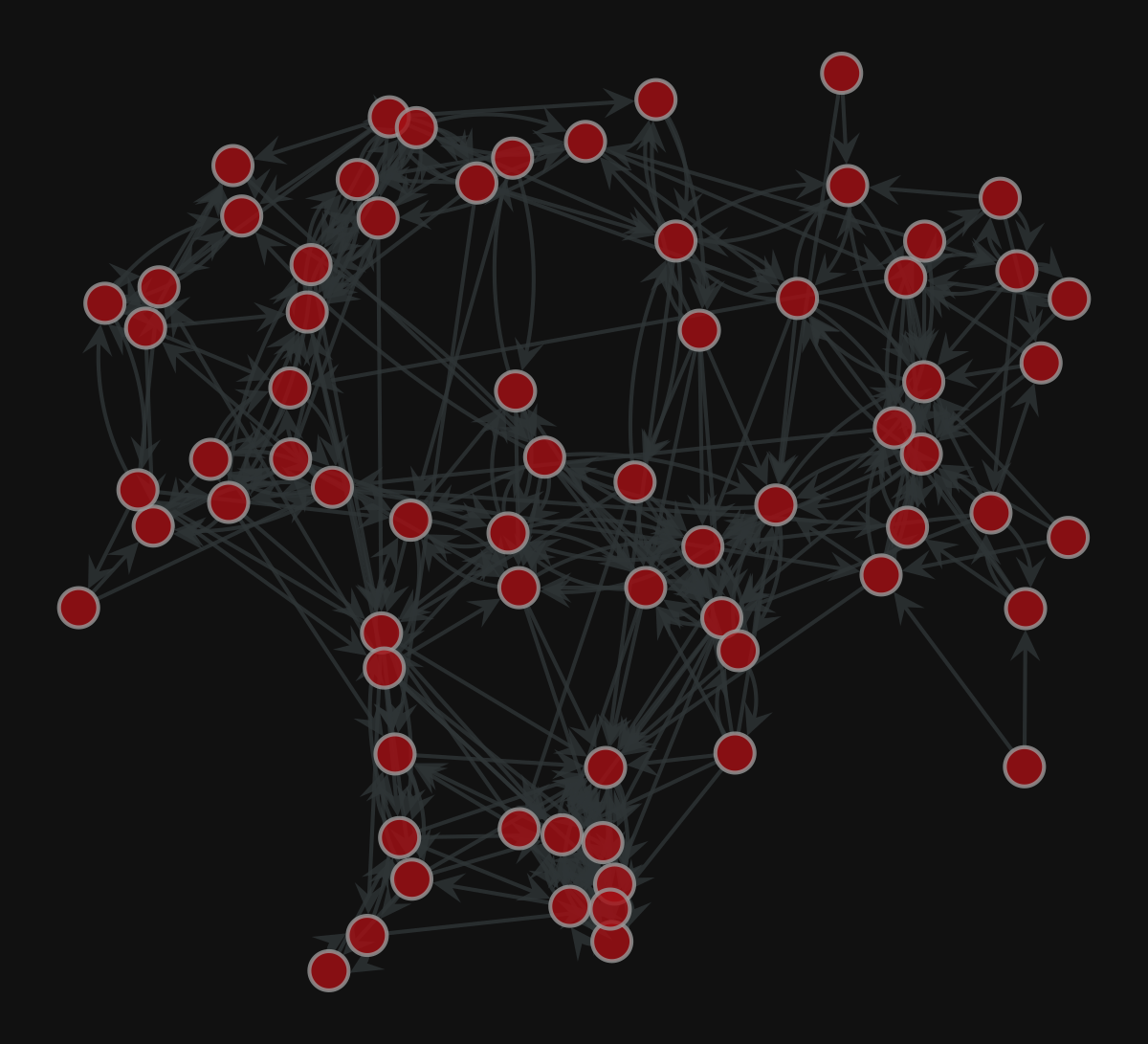<VertexPropertyMap object with value type 'vector<double>', for Graph 0x7f1ed41879d0, at 0x7f1ed411b9a0>

 1 2 3 4 5 6 7  pos = gt.random_layout(g) gt.graph_draw(g, pos=pos, edge_pen_width=g.edge_properties["weight"], bg_color="#111", )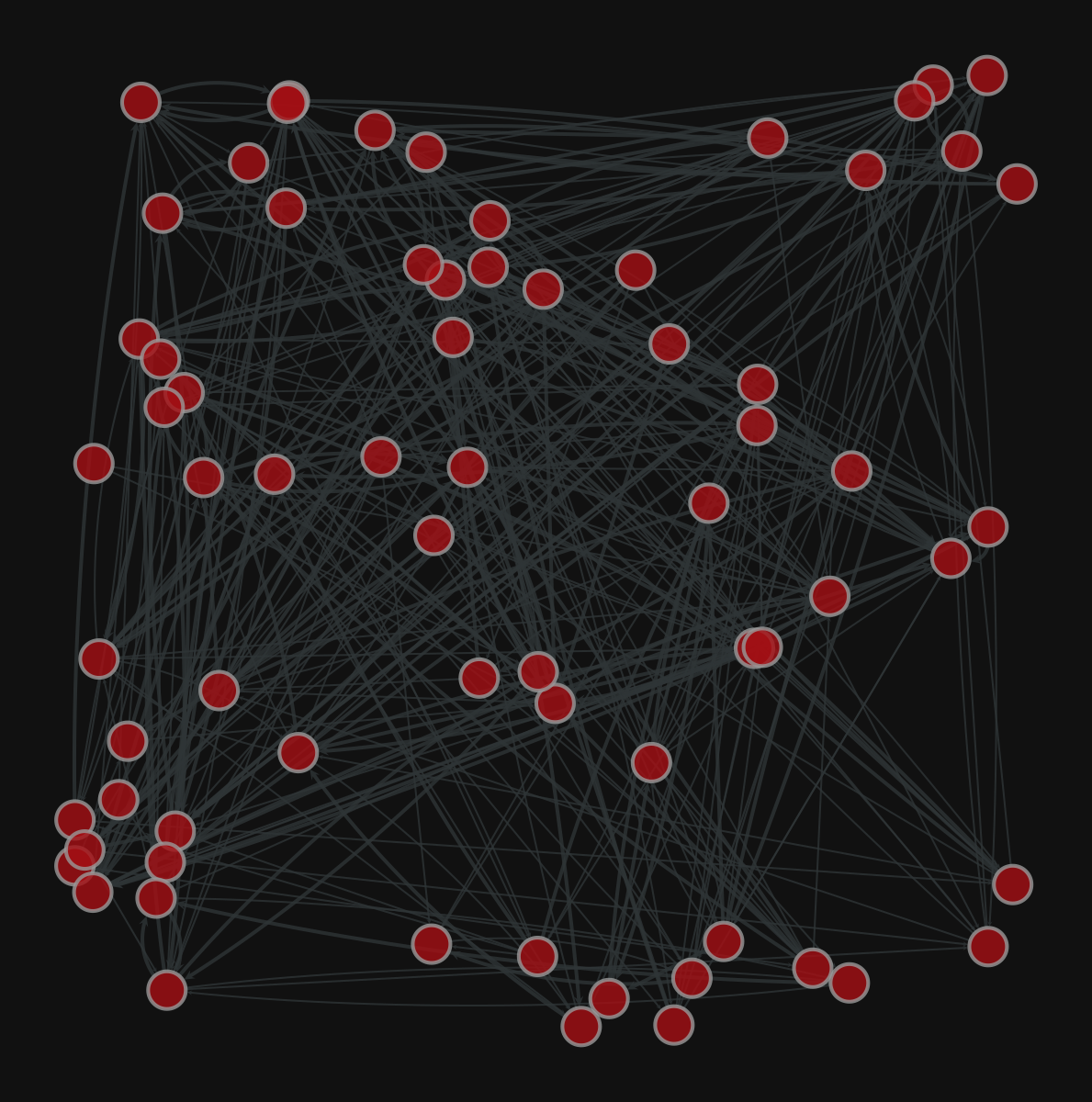<VertexPropertyMap object with value type 'vector<double>', for Graph 0x7f1ed41879d0, at 0x7f1ecf645ac0>


## Centrality computation

 1  gw = gt.GraphView(g, vfilt=gt.label_largest_component(g)) 

#### PageRank

 1  pr = gt.pagerank(g) 
 1 2  sns.displot(pr.a) plt.xlabel("PageRank") 
Text(0.5, 15.439999999999998, 'PageRank')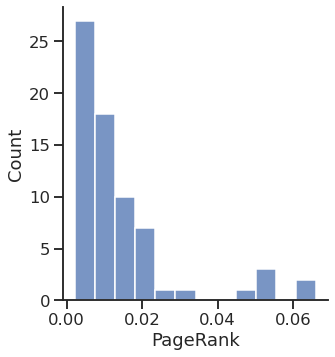#### Betweenness

 1  vertex_betweenness, edge_betweenness = gt.betweenness(g) 
 1 2  sns.displot(vertex_betweenness.a) plt.xlabel("Vertex betweenness") 
Text(0.5, 15.439999999999998, 'Vertex betweenness')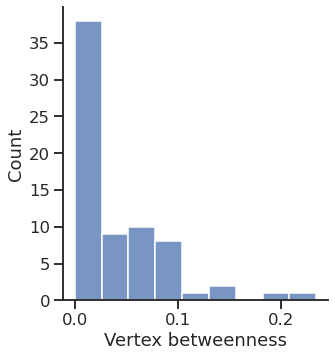1 2  sns.displot(edge_betweenness.a) plt.xlabel("Edge betweenness") 
Text(0.5, 15.439999999999998, 'Edge betweenness')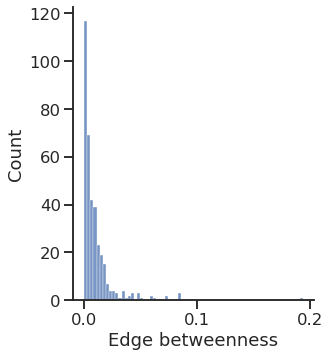1 2 3 4 5 6 7 8 9 10  gt.graph_draw(gw, pos=g.vp["_pos"], vertex_fill_color=pr, vorder=pr, edge_color=edge_betweenness, vertex_size=gt.prop_to_size(pr, mi=5, ma=15), vcmap=sns.color_palette("gist_heat", as_cmap=True), ecmap=sns.color_palette("rocket", as_cmap=True), edge_pen_width=g.edge_properties["weight"], bg_color="white", )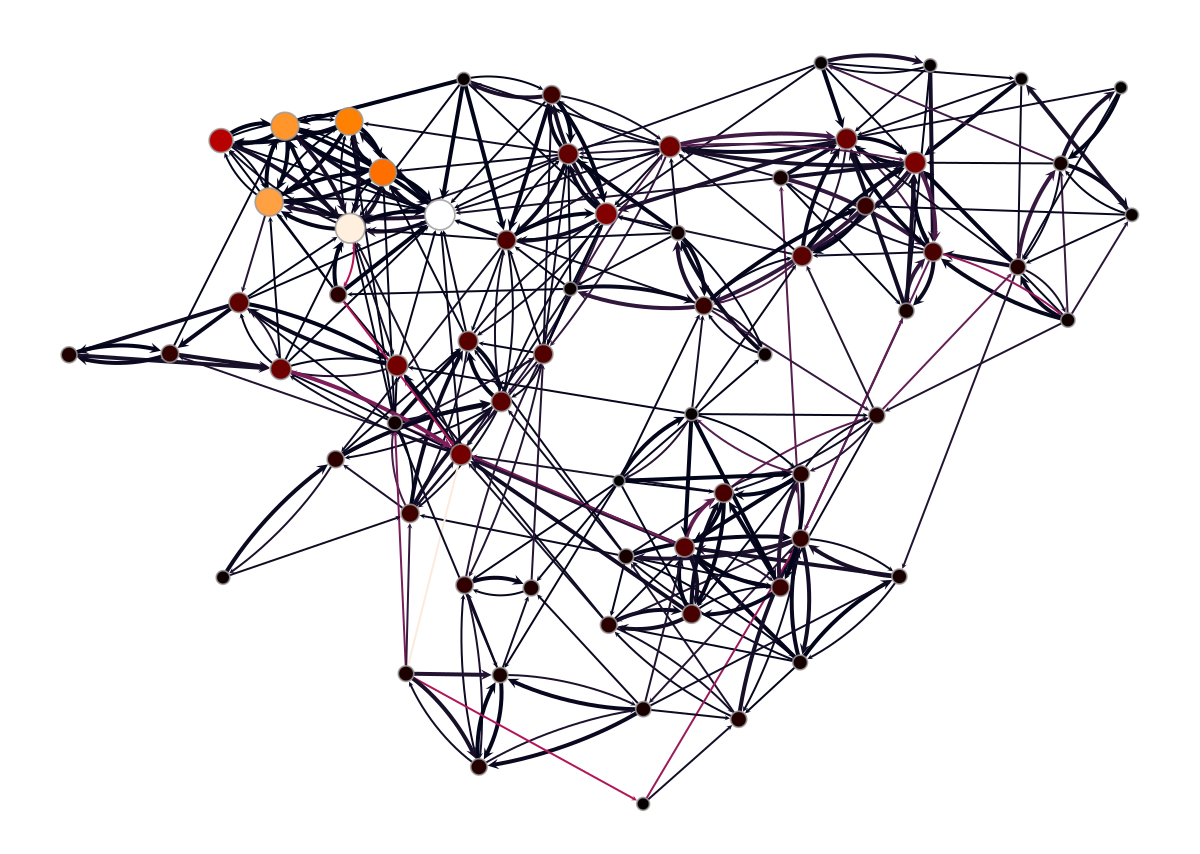<VertexPropertyMap object with value type 'vector<double>', for Graph 0x7f1ed4113fa0, at 0x7f1ed40db340>


## Inferring modular structure

 1 2  # state = gt.minimize_blockmodel_dl(g) state = gt.minimize_nested_blockmodel_dl(g) 
 1  state.draw()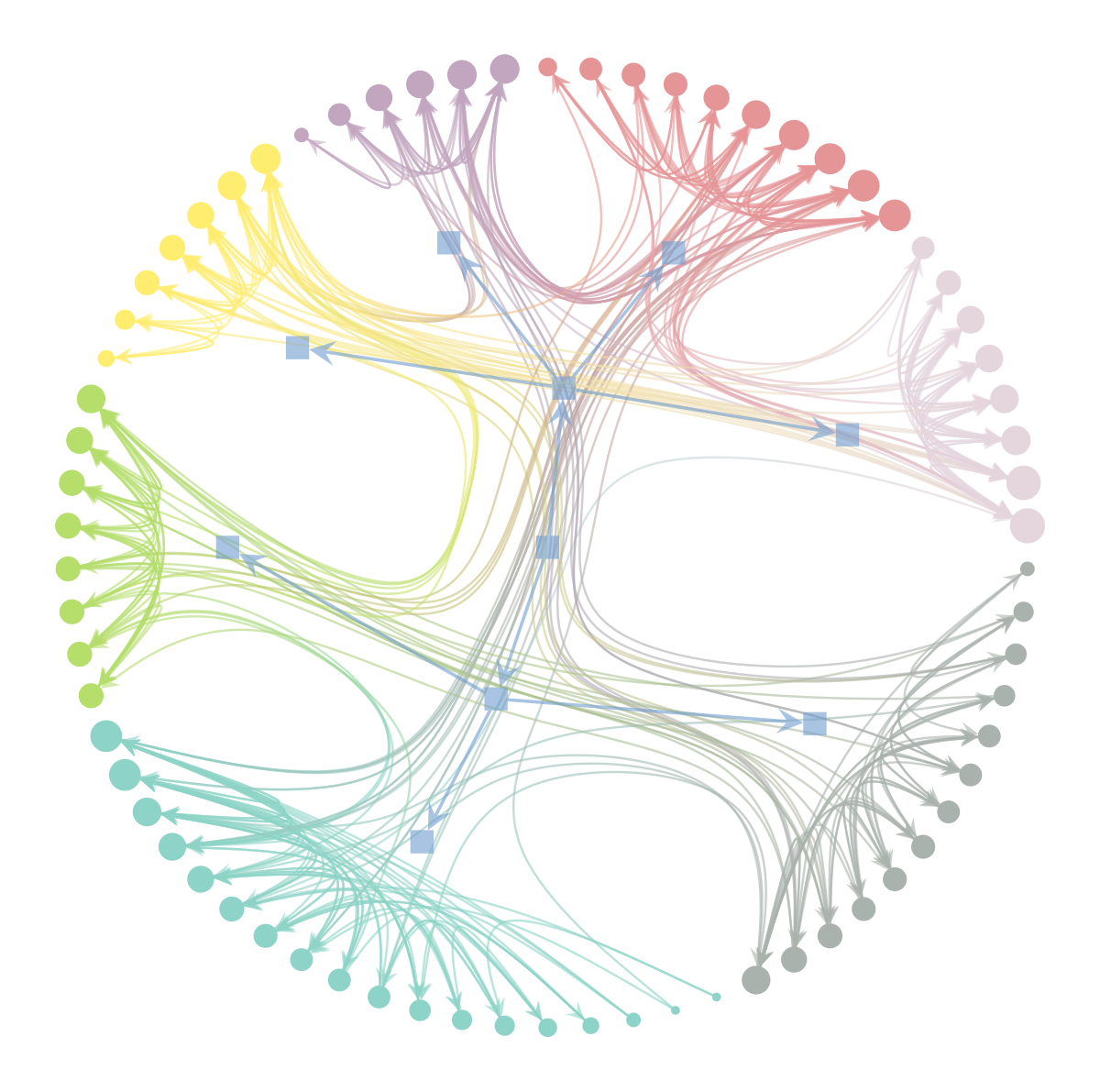(<VertexPropertyMap object with value type 'vector<double>', for Graph 0x7f1ed41879d0, at 0x7f1ecf7d77c0>,
<GraphView object, directed, with 80 vertices and 79 edges, edges filtered by (<EdgePropertyMap object with value type 'bool', for Graph 0x7f1ecf32dfa0, at 0x7f1ecf338040>, False), vertices filtered by (<VertexPropertyMap object with value type 'bool', for Graph 0x7f1ecf32dfa0, at 0x7f1ecf330ee0>, False), at 0x7f1ecf32dfa0>,
<VertexPropertyMap object with value type 'vector<double>', for Graph 0x7f1ecf32dfa0, at 0x7f1ecf330eb0>)

 1  levels = state.get_levels() 
  1 2 3 4 5 6 7 8 9 10 11  gt.graph_draw(g, pos=g.vp["_pos"], vertex_fill_color=levels.get_blocks(), edge_color=edge_betweenness, vertex_size=gt.prop_to_size(pr, mi=5, ma=15), vorder=pr, vcmap=sns.color_palette("tab10", as_cmap=True), ecmap=sns.color_palette("rocket", as_cmap=True), edge_pen_width=g.edge_properties["weight"], bg_color="white", )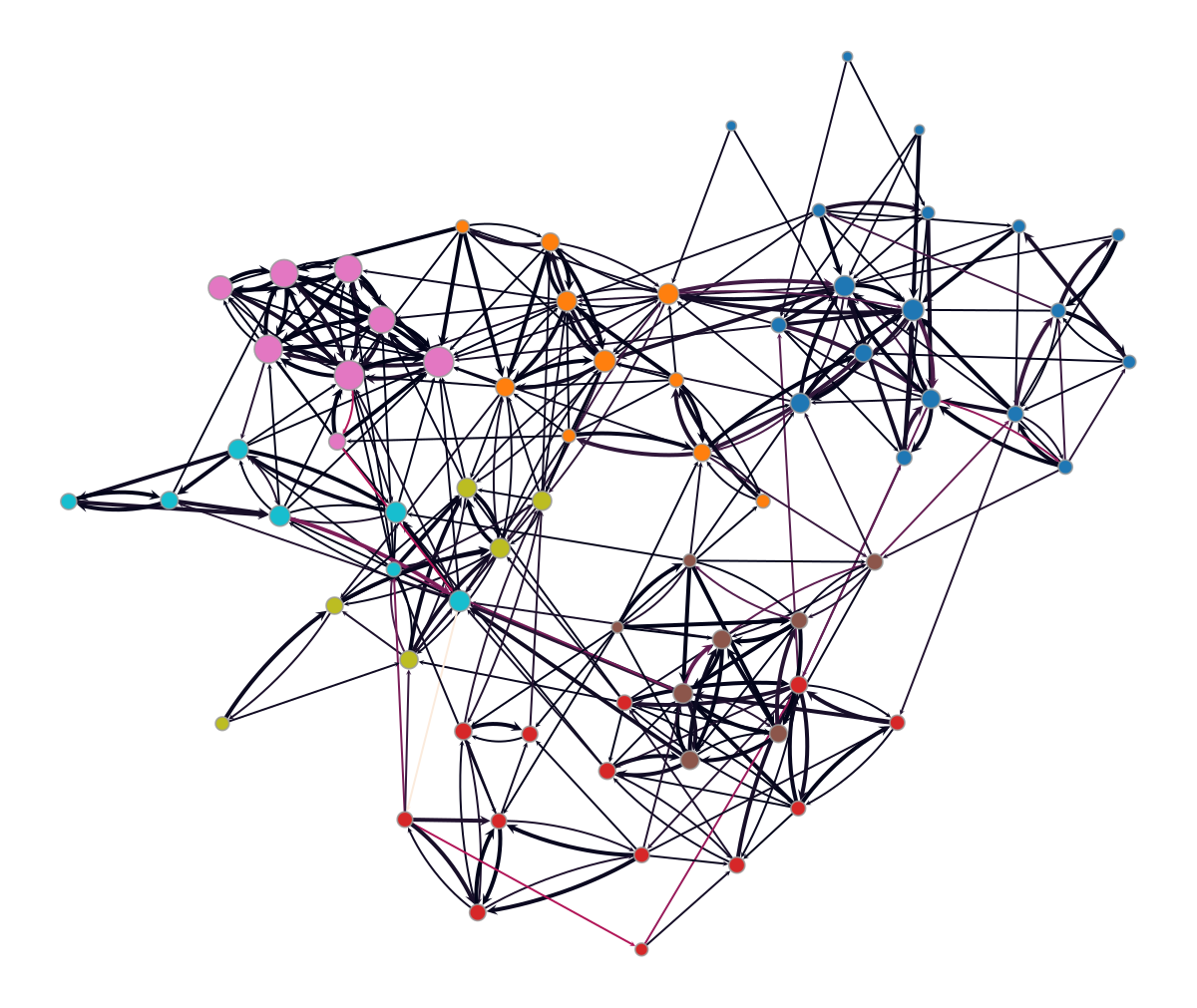<VertexPropertyMap object with value type 'vector<double>', for Graph 0x7f1ed41879d0, at 0x7f1ecf3c9310>


### Add some columns to our DataFrame

 1 2 3 4 5 6 7  df["PageRank"] = pr.a df["Betweenness"] = vertex_betweenness.a df["Block"] = levels.get_blocks().a df.head() 

DegreeStrengthPageRankBetweennessBlock
InOutInOut
026280.0038710.02491344
123340.0035020.00281944
224350.0036020.00564944
31261990.0205300.08132344
41352190.0228620.07907444

### Save the DataFrame

#### To CSV (Comma Separated Values)

 1  df.to_csv("dataframe.csv") 

#### To Excel

 1  df.to_excel("dataframe.xlsx") 

## PyTorch Geometric (PyG)

PyG is a library built upon PyTorch to easily write and train Graph Neural Networks (GNNs) for a wide range of applications related to structured data.

Library for Deep Learning on graphs

It provides a large collection of GNN and pooling layers

New layers can be created easily

It offers:

• Support for Heterogeneous and Temporal graphs
• Multi GPU-support
• DataPipe support
• Distributed graph learning via Quiver
• A large number of common benchmark datasets
• The GraphGym experiment manager

PyTorch Geometric documentation

### Introduction by example

Each network is described by an instance of torch_geometric.data.Data, which includes:

• data.x: node feature matrix, with shape [num_nodes, num_node_features]
• data.edge_index: edge list in COO format, with shape [2, num_edges] and type torch.long
• data.edge_attr: edge feature matrix, with shape [num_edges, num_edge_features]
• data.y: target to train against (may have arbitrary shape)
 1 2 3  import torch from torch_geometric.data import Data 

#### Node features

We do not have any node feature

We can use a constant for each node, e.g., $1$

 1 2 3 4 5 6 7  x = torch.ones(size=(g.num_vertices(), 1), dtype=torch.float32 ) display(x.shape) display(x.T) 
torch.Size([70, 1])

tensor([[1., 1., 1., 1., 1., 1., 1., 1., 1., 1., 1., 1., 1., 1., 1., 1., 1., 1.,
1., 1., 1., 1., 1., 1., 1., 1., 1., 1., 1., 1., 1., 1., 1., 1., 1., 1.,
1., 1., 1., 1., 1., 1., 1., 1., 1., 1., 1., 1., 1., 1., 1., 1., 1., 1.,
1., 1., 1., 1., 1., 1., 1., 1., 1., 1., 1., 1., 1., 1., 1., 1.]])


#### Connectivity matrix and edge attributes

  1 2 3 4 5 6 7 8 9 10  edge_index = torch.empty(size=(2, g.num_edges()), dtype=torch.long, ) display("edge_index", edge_index.shape) edge_attr = torch.empty(size=(g.num_edges(),), dtype=torch.float32, ) display("edge_attr", edge_attr.shape) 
'edge_index'

torch.Size([2, 366])

'edge_attr'

torch.Size()

  1 2 3 4 5 6 7 8 9 10  for i, (source, target, weight) in enumerate(g.iter_edges(eprops=[g.edge_properties["weight"]])): edge_index[0, i] = source edge_index[1, i] = target edge_attr[i] = weight display("edge_index", edge_index[:, :10]) display("edge_attr", edge_attr[:10]) 
'edge_index'

tensor([[ 0,  0,  0,  0,  0,  0,  1,  1,  1,  2],
[ 1,  2,  3,  4,  5,  6, 18,  3,  4,  0]])

'edge_attr'

tensor([1., 2., 2., 1., 1., 1., 1., 1., 2., 1.])


#### Create the Data instance

 1 2 3 4 5 6 7  network_data = Data( x=x, edge_index=edge_index, edge_attr=edge_attr, ) display(network_data) 
Data(x=[70, 1], edge_index=[2, 366], edge_attr=)


### Create the train and test set

For link prediction, we need positive (existent) and negative (non-existent) edges

We can use the RandomLinkSplit class, that does the negative sampling for us

  1 2 3 4 5 6 7 8 9 10 11  from torch_geometric.transforms import RandomLinkSplit transform = RandomLinkSplit(num_val=0, num_test=0.2, disjoint_train_ratio=0.2, split_labels=False, add_negative_train_samples=True, neg_sampling_ratio=1.0, is_undirected=False, ) train_data, _, test_data = transform(network_data) 
 1  display(train_data) 
Data(x=[70, 1], edge_index=[2, 235], edge_attr=, edge_label=, edge_label_index=[2, 116])

 1  display(test_data) 
Data(x=[70, 1], edge_index=[2, 293], edge_attr=, edge_label=, edge_label_index=[2, 146])


#### Create the model

Model architecture:

• 2x GINE convolutional layers
• 1x Multi-Layer Perceptron (MLP)

The GINE layers will compute the node embedding

We can build the edge embedding by, e.g., concatenating the source and target nodes’ embedding

The MLP will take the edge embeddings and return a probability for each

 1 2 3 4 5 6  from torch_geometric.nn import MLP class MLP(MLP): def __getitem__(self, item): return self.lins[item] 
  1 2 3 4 5 6 7 8 9 10 11 12 13 14 15 16 17 18 19 20 21 22 23 24 25 26 27 28 29 30  import torch.nn.functional as F from torch_geometric.nn import GINEConv from torch.nn import Sequential, Linear, ELU class GINEModel(torch.nn.Module): def __init__(self, in_channels, hidden_channels, out_channels, edge_dim): super().__init__() self.conv1 = GINEConv(nn=MLP([in_channels, hidden_channels, hidden_channels]), train_eps=False, edge_dim=edge_dim,) self.conv2 = GINEConv(nn=MLP([hidden_channels, hidden_channels, out_channels]), train_eps=False, edge_dim=edge_dim,) self.edge_regression = MLP(channel_list=[2 * out_channels, out_channels, 1], batch_norm=False, dropout=0.3) def forward(self, x, edge_index, target_edges): x = self.conv1(x=x, edge_index=edge_index, edge_attr=edge_attr) x = F.relu(x) x = self.conv2(x=x, edge_index=edge_index, edge_attr=edge_attr) x = F.relu(x) x = torch.hstack(( x[target_edges[0, :]], x[target_edges[1, :]], )) x = self.edge_regression(x) return x 

#### Let’s create the model instance

 1 2 3 4 5 6 7  model = GINEModel(in_channels=network_data.x.shape, hidden_channels=20, out_channels=20, edge_dim=network_data.edge_attr.shape, ) display(model) 
GINEModel(
(conv1): GINEConv(nn=MLP(1, 20, 20))
(conv2): GINEConv(nn=MLP(20, 20, 20))
(edge_regression): MLP(40, 20, 1)
)


#### Create the optimizer and loss instances

 1 2 3 4 5  optimizer = torch.optim.Adam(params=model.parameters(), lr=0.005, weight_decay=1e-4) criterion = torch.nn.BCEWithLogitsLoss() # criterion = torch.nn.MSELoss() epoch = 0 

#### Define the train function and train the model

  1 2 3 4 5 6 7 8 9 10  def train(): model.train() optimizer.zero_grad() edge_probability = model(train_data.x, train_data.edge_index, train_data.edge_label_index).squeeze() loss = criterion(edge_probability, train_data.edge_label) loss.backward() optimizer.step() return loss 

#### Define the test function

 1 2 3 4 5 6 7 8  @torch.no_grad() def test(): model.eval() edge_probability = model(test_data.x, test_data.edge_index, test_data.edge_label_index).squeeze() edge_probability = torch.sigmoid(edge_probability) return edge_probability, test_data.edge_label 

### Training and testing

 1 2 3 4 5 6 7 8 9  from tqdm import tqdm for _ in tqdm(range(2001)): loss = train() if epoch % 500 == 0: display(f'Epoch: {epoch:03d}, Loss: {loss:.4f}') epoch += 1 
 24%|███████████████████████████████████████████████████████████████████▏                                                                                                                                                                                                               | 489/2001 [00:02<00:05, 262.96it/s]

'Epoch: 2500, Loss: 0.0881'

50%|████████████████████████████████████████████████████████████████████████████████████████████████████████████████████████████████████████▏                                                                                                                                          | 991/2001 [00:04<00:04, 211.13it/s]

'Epoch: 3000, Loss: 0.1207'

75%|████████████████████████████████████████████████████████████████████████████████████████████████████████████████████████████████████████████████████████████████████████████████████████████████████████████▋                                                                     | 1495/2001 [00:06<00:01, 271.06it/s]

'Epoch: 3500, Loss: 0.0798'

99%|██████████████████████████████████████████████████████████████████████████████████████████████████████████████████████████████████████████████████████████████████████████████████████████████████████████████████████████████████████████████████████████████████████████████▏   | 1973/2001 [00:08<00:00, 291.01it/s]

'Epoch: 4000, Loss: 0.0769'

100%|██████████████████████████████████████████████████████████████████████████████████████████████████████████████████████████████████████████████████████████████████████████████████████████████████████████████████████████████████████████████████████████████████████████████████| 2001/2001 [00:08<00:00, 241.67it/s]

 1  edge_probability, test_y = test() 

### Evaluate the model performance

The torchmetrics package provides many performance metrics for various tasks

It is inspired by scikit-learn’s metrics subpackage

 1 2 3 4 5 6 7 8  from torchmetrics import Accuracy, AUROC accuracy = Accuracy(threshold=0.5) auroc = AUROC() display("Accuracy", accuracy(edge_probability, test_y.to(torch.int)).item()) display("AUROC", auroc(edge_probability, test_y.to(torch.int)).item()) 
'Accuracy'

0.6095890402793884

'AUROC'

0.6100582480430603##### Marco Grassia
###### Research Fellow · Network Science and Machine Learning

Research Fellow in Network Science and Machine Learning at University of Catania